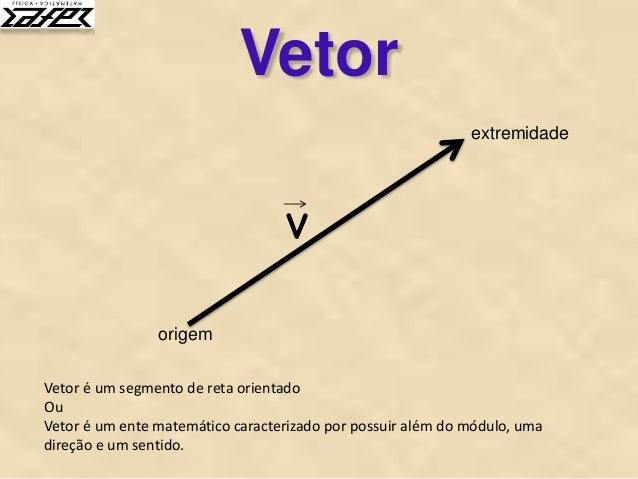Successfully reported this slideshow.Upcoming SlideShare
×

Vetores

6,014 views

Published on

Rápida introdução ao estudo dos vetores como pré-requisito para a cinemática vetorial

Published in: Education
• Full Name
Comment goes here.

Are you sure you want to Yes No• Be the first to comment

Vetores

1. 1. Vetor v origem extremidade Vetor é um segmento de reta orientado Ou Vetor é um ente matemático caracterizado por possuir além do módulo, uma direção e um sentido.
2. 2. Vetor v O módulo do vetor é representado geometricamente pelo comprimento do segmento de reta. Módulo A notação módulo do vetor é | v | ou simplesmente v (sem a setinha em cima da letra) Nesse caso o módulo do vetor é de 8 unidades
3. 3. Vetor v Se tomarmos o quadradinho como unidade, podemos dizer que: Módulo A notação módulo do vetor é | v | ou simplesmente v (sem a setinha em cima da letra) o módulo do vetor v é de 8 unidades u o módulo do vetor v é de 5 unidades
4. 4. Vetor v Direção A direção do vetor é dada pelo ângulo que ele faz com a horizontal. q
5. 5. Vetor v Direção O vetor pode ser 45 vertical horizontal inclinado u w
6. 6. Vetor v Sentido O sentido do vetor é determinado pela seta.
7. 7. Vetor Sentido O sentido do vetor pode ser De baixo para cima De cima para baixo Da direita para a esquerda Da esquerda para a direita Se for inclinado, do sudoeste para o nordeste, por exemplo
8. 8. Vetor Adição de vetores Caso 1: Vetores de mesma direção e mesmo sentido Caso 1: Vetores de mesma direção e mesmo sentido v u s Módulo do vetor v v = 4 unid. Módulo do vetor u u = 6 unid. S = v + u S = 4 + 6 S = 10 unid.
9. 9. Vetor Adição de vetores Caso 2: Vetores de mesma direção e sentido oposto Observe que o vetor resultante tem o sentido igual ao do vetor de maior módulo. Módulo do vetor a a = - 4 unid. Módulo do vetor b b = 6 unid. S = a + b S = - 4 + 6 S = 2 unid. Módulo do vetor c c = 4 unid. Módulo do vetor d d = 6 unid. S = c + d S = 4 + (- 6) S = -2 unid. a b c d s s
10. 10. Vetor Adição de vetores Caso 2: Vetores de mesma direção , sentido oposto e mesmo módulo Observe que os vetores se anulam, o que equivale dizer que o vetor resultante tem módulo zero ou ainda que é um vetor nulo. Módulo do vetor a a = - 6 unid. Módulo do vetor b b = 6 unid. S = a + b S = - 6 + 6 S = 0 unid. b a
11. 11. Vetor Adição de vetores Caso 3: Vetores ortogonais*
12. 12. Vetor Adição de vetores Caso 3: Vetores ortogonais*
13. 13. Vetor Adição de vetores Caso 3: Vetores ortogonais módulo do vetor soma a b s a b s s é a hipotenusa e a e b são catetos Pelo teorema de Pitágoras, temos: Se a = 8 unid e b = 6 unid, temos s2 = 82 + 62 s2 = 64 + 36 s2 = 100 s = 100 s = 10 unid. s2 = a2 + b2
14. 14. Vetor Decomposição de vetores Componentes horizontal e vertical x y
15. 15. Vetor Decomposição de vetores Componentes horizontal e vertical x y vx v vy vy v vx
16. 16. Vetor Decomposição de vetores Componentes horizontal e vertical x y vy vx v q q vy v vx Módulo de vy v é hipotenusa do triângulo vy é cateto oposto a q vx é cateto adjacente a q 𝑠𝑒𝑛 𝜃 = 𝑣 𝑦 𝑣 𝑣. 𝑠𝑒𝑛 𝜃 = 𝑣 𝑦 𝒗 𝒚 = 𝒗. 𝒔𝒆𝒏 𝜽
17. 17. Vetor Decomposição de vetores Componentes horizontal e vertical x y vy vx v q q vy v vx Módulo de vx v é hipotenusa do triângulo vy é cateto oposto a q vx é cateto adjacente a q 𝑐𝑜𝑠 𝜃 = 𝑣 𝑥 𝑣 𝑣. 𝑐𝑜𝑠 𝜃 = 𝑣 𝑥 𝒗 𝒙 = 𝒗. 𝒄𝒐𝒔 𝜽